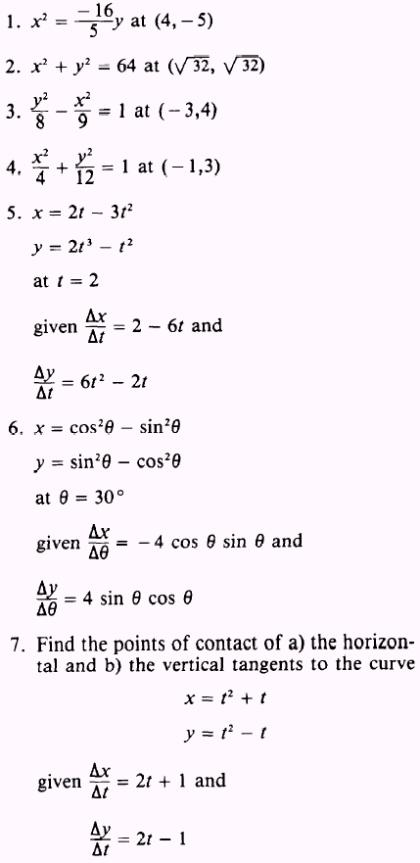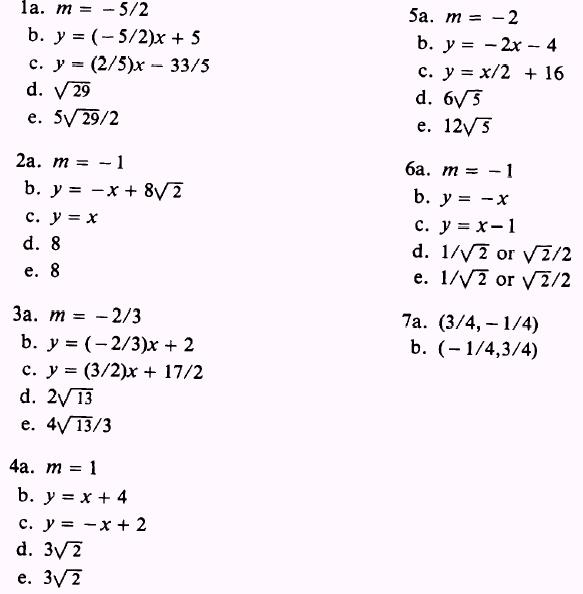Custom SearchADDITIONAL PRACTICE PROBLEMS Find a) the slope, b) equation of the tangent line, c) equation of the normal line, d) length of the tangent, and e) length of the normal, in problems 1 through 6, at the given points.ANSWERS TO ADDITONAL PRACTICE PROBLEMS